# Fourier-Bessel series

(Redirected from Fourier–Bessel series)

The expansion of a functionin a series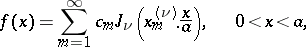(*)

whereis a function given on the interval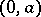,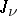is the Bessel function of order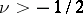(cf. Bessel functions), and the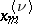are the positive zeros oftaken in increasing order; the coefficients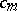have the following values: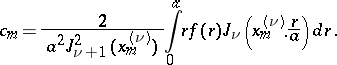Ifis a piecewise-continuous function given on an intervaland if the integralthen the Fourier–Bessel series converges and its sum is equal toat each interior pointofat whichlocally has bounded variation.

How to Cite This Entry:
Fourier–Bessel series. Encyclopedia of Mathematics. URL: http://www.encyclopediaofmath.org/index.php?title=Fourier%E2%80%93Bessel_series&oldid=22440